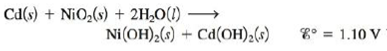# The overall reaction and standard cell potential at 25°C for the rechargeable nickel-cadmium alkaline battery is For every mole of Cd consumed in the cell, what is the maximum useful work that can be obtained at standard conditions?### Chemistry: An Atoms First Approach

2nd Edition
Steven S. Zumdahl + 1 other
Publisher: Cengage Learning
ISBN: 9781305079243

#### Solutions

Chapter
Section### Chemistry: An Atoms First Approach

2nd Edition
Steven S. Zumdahl + 1 other
Publisher: Cengage Learning
ISBN: 9781305079243
Chapter 17, Problem 126AE
Textbook Problem
26 views

## The overall reaction and standard cell potential at 25°C for the rechargeable nickel-cadmium alkaline battery isFor every mole of Cd consumed in the cell, what is the maximum useful work that can be obtained at standard conditions?

Interpretation Introduction

Interpretation:

The overall reaction and standard cell potential at 25°C for the rechargeable Nickel-Cadmium alkaline battery is given. The maximum useful work that can be obtained for every mole of Cadmium consumed at standard conditions is to be calculated.

Concept introduction:

Gibbs free energy is basically the maximum amount of non-expansion work done. Therefore, it is represented as,

Wmax=ΔG°

The maximum amount of work that is done by an electrochemical cell is given as,

Wmax=nFE°cell

The relationship between Gibbs free energy change and equilibrium constant is given by the formula,

ΔG°=nFE°cell

The work is done by the system over the surroundings; therefore the work is taken as a negative term.

To determine: The maximum useful work that can be obtained for every mole of Cadmium consumed at standard conditions.

### Explanation of Solution

Given,

The value of E°cell is 1.10V .

The reaction taking place at cathode is,

NiO2+2H2O+2eNi(OH)2+2OH

The reaction taking place at anode is,

Cd+2OHCd(OH)2+2e

The overall reaction is,

Cd(s)+NiO2(s)+2H2O(l)Ni(OH)2(s)+Cd(OH)2(s)

The reaction involves the transfer of 2 moles of electrons.

The relationship between Gibbs free energy change and cell potential is given by the formula,

ΔG°=nFE°cell

Where,

• ΔG° is the Gibbs free energy change at the standard conditions.
• n is the number of moles of electrons that are involved in the reaction

### Still sussing out bartleby?

Check out a sample textbook solution.

See a sample solution

#### The Solution to Your Study Problems

Bartleby provides explanations to thousands of textbook problems written by our experts, many with advanced degrees!

Get Started

Find more solutions based on key concepts
Describe how Dalton obtained relative atomic weights.

General Chemistry - Standalone book (MindTap Course List)

After about 50 years of age, bones begin to lose dens.

Nutrition: Concepts and Controversies - Standalone book (MindTap Course List)

It has been suggested dial rotating cylinders about 20 km in length and 8 km in diameter be placed in space and...

Physics for Scientists and Engineers, Technology Update (No access codes included)

What is the difference between sensible and nonsensible heat?

Oceanography: An Invitation To Marine Science, Loose-leaf Versin

Why do astronomers now label Pluto a dwarf planet?

Foundations of Astronomy (MindTap Course List)